### Unity Voxel Tutorial Part 3: Perlin noise for terrain

#### Hello everyone following the voxel tutorial, it's been a long time since an update. In this time I've written a new updated tutorial on voxel terrain that supports infinite terrain and saving/loading of chunks. Try it out on my new site: AlexStv.com

Welcome to part three of the voxel tutorial. We'll be setting up the 2d game with some perlin noise to give the terrain some shape.

We'll get started by using perlin noise to create some varied terrain and caves from our blocks. Start by increasing the size of the block array to 96x128 so that we have some more room to work with. This is defined in the GenTerrain function. Also take away the old code that we used to generate terrain, the one that gave us 5 rows of blocks.

```void GenTerrain(){
blocks=new byte[96,128];

for(int px=0;px<blocks.GetLength(0);px++){

for(int py=0;py<blocks.GetLength(1);py++){

}
}
}
```

To use perlin noise I like to use a separate function to call the Mathf.PerlinNoise unity function so that I can include things like scale and apply exponents with the parameters of my function. Here's that function:
```int Noise (int x, int y, float scale, float mag, float exp){

return (int) (Mathf.Pow ((Mathf.PerlinNoise(x/scale,y/scale)*mag),(exp) ));

}
```

Perlin noise is an algorithm created by Ken Perlin to create gradient noise. Other people can explain it much better than me: This is a good source. But all you really need to know is that it returns values between 1 and 0 based on the values you enter. It can be used for numbers of dimentions far beyond what we need but the Unity function only takes two. For now that will be fine because this example is 2d.

What the function above does is it takes coordinates for x and y to sample for noise, then it calls the perlin noise function with those divided by scale. Because perlin noise isn't random but bases itself on the coordinates supplied then the closer those coordinates are to each other the more similar the values it returns. So when we divide the coordinates by a number they end up as smaller numbers closer to each other. (1,0) and (2,0) might return 0.5 and 0.3 respectively but if we divide them by two calling perlin noise for (0.5,0) and (1,0) instead the numbers might be 0.4 and 0.5. This will be more clear once we apply it to the terrain.

Then we take the value we get from perlin noise and multiply it by the magnitude "mag" because perlin noise returns a value between 0 and 1 and we are going to want noise that creates hills that vary in height by larger sizes like between 0 and 10. Then we take the result and put it to the power of the exponent "exp". This is useful for mountains and things. Lastly we convert the float returned into an int.

We'll apply this to the GenTerrain function column by column. By getting a number for perlin noise in the first loop (for each x) and then using that number in the y loop as the height of the terrain:

```void GenTerrain(){
blocks=new byte[96,128];

for(int px=0;px<blocks.GetLength(0);px++){
int stone= Noise(px,0, 80,15,1);
stone+= Noise(px,0, 50,30,1);
stone+= Noise(px,0, 10,10,1);
stone+=75;

int dirt = Noise(px,0, 100,35,1);
dirt+= Noise(px,0, 50,30,1);
dirt+=75;

for(int py=0;py<blocks.GetLength(1);py++){
if(py<stone){
blocks[px, py]=1;

} else if(py<dirt) {
blocks[px,py]=2;
}

}
}
}
```

We create a stone int and a dirt int and using a few layers of perlin noise they get more textured values. Because this is essentially a 1d heightmap we only need x and the y variable can be used just to sample from a different area to make sure the results aren't the same. You can see the stone is three noise layers with different values.

Layer 1:
```int stone= Noise(px,0, 80,15,1);
```

Layer one has a scale of 80 making it quite smooth with large rolling hills, the magnitude is 15 so the hills are at most 15 high (but in practice they're usually around 12 at the most) and at the least 0 and the exponent is 1 so no change is applied exponentially.

Layer 2:
```stone+= Noise(px,0, 50,30,1);
```

The next layer has a smaller scale so it's more choppy (but still quite tame) and has a larger magnitude so a higher max height. This ends up being the most prominent layer making the hills.

Layer 3:
```stone+= Noise(px,0, 10,10,1);
```

The third layer has an even smaller scale so it's even noisier but it's magnitude is 10 so its max height is lower, it's mostly for adding some small noise to the stone to make it look more natural. Lastly we add 75 to the stone to raise it up.

The dirt layer has to be mostly higher than the stone so the magnitudes here are higher but the scales are 100 and 50 which gives us rolling hills with little noise. Again we add 75 to raise it up.

The result is a noisy stone layer with highs and lows and a smooth dirt layer on top that's usually higher than the stone layer but sometimes the stone sticks out. You could also change the y value to offset the location of the noise sample. This is applied in the y loop where we change all blocks with a y below the stone int to stone and if they're higher than the stone (else) we check if y is below the dirt in and if so change the blocks to dirt.Stone and dirt noise.
Also with noise we will add caves and spots of dirt in the stone. Surprisingly this is simpler than the dirt and stone layers. What we do is that in the if function for creating stone to a certain height we add another two ifs for caves and dirt so if the block is made into stone check to see if it should be a cave or a dirt spot instead. Here we'll use both x and y because the noise should be 2d.

```void GenTerrain(){
blocks=new byte[96,128];

for(int px=0;px<blocks.GetLength(0);px++){
int stone= Noise(px,0, 80,15,1);
stone+= Noise(px,0, 50,30,1);
stone+= Noise(px,0, 10,10,1);
stone+=75;

print(stone);

int dirt = Noise(px,0, 100f,35,1);
dirt+= Noise(px,100, 50,30,1);
dirt+=75;

for(int py=0;py<blocks.GetLength(1);py++){
if(py<stone){
blocks[px, py]=1;

//The next three lines make dirt spots in random places
if(Noise(px,py,12,16,1)>10){
blocks[px,py]=2;

}

//The next three lines remove dirt and rock to make caves in certain places
if(Noise(px,py*2,16,14,1)>10){ //Caves
blocks[px,py]=0;

}

} else if(py<dirt) {
blocks[px,py]=2;
}

}
}
}
```

So you see inside the stone if ( if(py<stone) ) we also have an if that compares noise with 10 so if the noise we return is larger than 10 it turns the block to dirt instead of stone. The magnitude of the noise value is 16 so it reruns a over 10 only a little of the time and the scale is fairly low so the spots are pretty small and frequent. We're using x and y here and running the if for every block so the dirt is distributed through the whole array.

After that we add caves with a similar function but we multiply y by two to stretch out the caves so they are wider than they are tall and we use a larger scale to make larger less frequent caves and the magnitude is lower to reduce the size of the caves that was increased by the scale.Now you should get caves and dirt spots.
The caves and spots are pretty evenly distributed, I like to use y to change the scale and magnitude for both to make caves more likely and large the lower you go and the opposite for dirt spots but that's all taste. You can also use similar functions to add things like ores and things.

Thanks for reading part 3, let me know about any problems you find or feedback you think of. Follow me on twitter (@STV_Alex) or G+ to get updated when I post part four. It should be out very shortly, part three was actually going to include it but I decided to split them up, anyway in that one we'll be destroying and placing blocks!

Edit: Thanks again to Taryndactyl! Taryndactyl's post: Link

Part 4

1.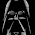Awsome, works well. But gee if I make a single little error when typing somewhere or it turns out quite wrong, its quite funny when I make a simple mistake and spend and hour going back over it all to find out what I didn't do lol.

1.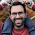Mind if I ask what mistake it was? Maybe I could clear it up a bit for anyone else following the tutorial.

2.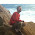Thanks Alex for this awesome work, it really motivated me to try i harder.
Just one question arises in me while i'm working on this- the perlin noise is not really random, as it generates always the same output from the same input(and here we are giving always the same input). Could you give me some hint on how to work on your code to give it an random appearance?
Thank you very much once again for you great effort! ;-)

1.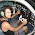You could try creating a Public variable that acts like a seed for example:
public float NoiseSeed=0;

and then use it like this:
dirt+= Noise(px,NoiseSeed, 100,35,1);
if you change Y, acting like a seed you will go over different parts of the perlin noise function and that will give you different results on the same spot.

2.Going into this a little bit more, a good seed to use is the current time.

3.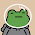How do I actually get it to generate something all it does its generate numbers but no blocks or anything.

4.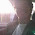Tks man! very cool! the result is great

5.How would I go about implementing chunks and loading / unloading them ? I would like it to be an infinite terrain so we cannot store all the chunk data in one array

1.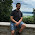That's actually implemented later in this tutorial, and it's fairly simple.

What I did is I just created a World GameObject that had a script attached which would instantiate a chunk prefab and then store it as an item in a two-dimensional array. From there I would change its position, biome, etc.

Hope this helped you.

6.Great tutorial so far. I think you should split the return line in the noise function into 4 or so lines to make it easier to read/understand/explain. I had to do that before I could tell what was going on.

float height = Mathf.PerlinNoise(x/scale,y/scale);
height = height * magnitude;
height = Mathf.Pow (height, exp);
return (int) height;

7.For those interested by going in depth with what perlin noise is, there's a great explanation here :
http://freespace.virgin.net/hugo.elias/models/m_perlin.htm

and to go more in peth , since perlin noise is a heavy concept to grasp :
linear interpolation : http://en.wikipedia.org/wiki/Linear_interpolation
to grasp linear interpolation formula, you can tackle it with an angle approach , tan(alpha) :
http://en.wikipedia.org/wiki/Triangle#Sine.2C_cosine_and_tangent

Potentially after that, you can change the basic random formula used in order to have many different effects.
I hope it will helps people like me who lacks some maths and are willingly trying to go farther than this great tutorial ! Thanks a lot M. Stavrinou !

8.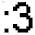caves are not generating for me

9.Hay so i have 2 questions... well more like 3 so first off do you see any of these newer posts? You dont reply as much. 2nd on my list is is there a way i can implement the marching square algorithm into this so it looks more smooth? and lastly is do you want me to make a youtube video saying the same stuff because i know that if i can see some one doing this while saying whats going on it will help me alot more as well as get this post viewed more and it would be fun to turn this into a youtube tutorial!

1.Oh and one more question how do i add a grass layer or is there a way to turn the dirt that hits air into grass?

10.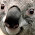Hey ! I don't know if you'll read it but maybe some one else can help me.

How would i limit the height the terrain gets generated up to ?

11.It doesn't spawn random terrain the deposits of dirt and caves seem to generate in the same area every time.